# Connors RSI — What Is It? (Trading Strategy and Indicator Backtest)

Last Updated on June 19, 2022 by Quantified Trading

Connors RSI including backtest

The traditional RSI indicator has been a great tool for technical analysis and trading, but there have been some efforts by traders to improve on it. One of such improvements gave rise to the Connors RSI, which can be an invaluable tool for traders when applied correctly — not only does it allow you to construct intra-day trading strategies with a high probability of success, but it also reduces your risk potential. But what exactly is the Connors RSI indicator? We explain Connors RSI and compare it to both Welles Wilder’s RSI and Cutler’s RSI. Finally, we make some backtests and potential trading strategies based on the indicator.

Often referred to as CRSI, the Connors RSI indicator is a momentum-based oscillator that tries to improve on the original 14-period RSI indicator developed by Welles Wilder. Unlike the original 14-period RSI indicator, the CRSI uses 2 periods as its lookback input parameter and combines the momentum measurement of RSI with components that measure the duration of the trend and the magnitude of the price change to create a more reliable short-term RSI indicator. The CRSI indicator was developed by Larry Connors to create a variation of RSI that would adapt better to short-term changes in the market.

## What is Connors’ RSI indicator?

The Connors RSI indicator, commonly referred to as CRSI, is a momentum-based oscillator that tries to improve on the traditional 14-period RSI indicator developed by J. Welles Wilder. Developed by Larry Connors, the CRSI was an attempt to create a variation of the original RSI that would adapt better to short-term changes in the market.

Unlike the original 14-period RSI indicator, the CRSI uses 2 periods as its lookback input parameter and combines the momentum measurement of RSI with components that measure the duration of the trend and the magnitude of the price change to create a more reliable short-term RSI indicator. The Connors RSI defines an overbought level that reaches a minimum of 90, or 95 in certain cases.

The Connors RSI indicator is actually a composite of three separate components — The RSI, UpDown Length, and Rate-of-Change — but Wilder’s traditional RSI plays an integral role in the final indicator. In fact, Wilder’s original RSI is used in two of the indicator’s three components.

The three components combine to form a momentum oscillator whose values range between 0 and 100. As with the traditional RSI, it is used to identify short-term overbought and oversold conditions, but unlike the traditional one, it works better in shorter trading timeframes. As a momentum oscillator, it also shows the strength and velocity of price movements.

Since the traditional 14-period RSI indicator developed by Welles Wilder reacts too slowly to be useful for short-term trading, Connors Research sought to improve on it by making the new RSI more suitable for shorter timeframes.

Initially, they developed a 2-period RSI strategy, which used a 2-period RSI and moved overbought/oversold levels to 90 and 10, but this approach still resulted in more false signals than desired. So, the team then created a composite indicator that combines the momentum measurement of RSI with components that measure the duration of the trend and the magnitude of the price change to create a more reliable short-term RSI indicator. Traders use the readings to gauge the momentum of price movements.

When attached to the chart, the indicator is usually displayed in the indicator box under the price chart. The indicator line moves between the 0 and 100 readings, and its overbought level is set to 90 and above, while the oversold level is set to 10 and below. Thus, a reading of 10 or lower signifies an oversold market, while a reading of 90 and above signifies an overbought market. The indicator can also show divergence from the price movements when the price movement and the indicator movement are not synchronized, but the signals are short-lived since it is used in short timeframes.

## What is the formula for Connors RSI?

The formula for calculating Connors RSI is quite complicated because the indicator has three components, which must first be calculated individually. But the final step is calculated by taking the average of its three components. The formula for that is given as follows:

Connors RSI (3,2,100) = [RSI(3) + RSI(Streak,2) + PercentRank(100)] / 3

This shows that it is the average of the 3 components of the indicator. Let’s now look at how the components are calculated.

### How is Connors RSI calculated?

The 3 components of the Connors RSI indicator are as follows:

1. Relative Strength Index (RSI)
2. Up/Down Length (Market Streak Value)
3. Rate of Change (ROC) or magnitude of price change

Relative Strength Index

The first component is a simple 3-period RSI of price. This component measures price momentum on a scale of 0-100. It is calculated just like the traditional RSI, but here, the averaging period is 3. The calculation is as follows:

Upward change (U) = Closenow — Closeprevious while D =0

Downward change (D) = Closeprevious — Closenow while U =0

Both U and D = 0, if both previous and current closes are the same.

Next, the relative strength factor (RS) is calculated with this formula:

RS = SMMA (U, 3)/SMMA (D, 3)

Where:

SMMA (U, 3) = 3-period smoothed moving average of U

SMMA (D, 3) = 3-period smoothed moving average of D

The RSI is then calculated as:

RSI = 100 — [100/(1 + RS)]

Up/Down Streak Length

This component is a 2-period RSI of the up/down streak length, which measures the duration of the trend. It is essentially the number of days in a row that the security’s closing price has been higher (up) or lower (down) than the previous day’s close. The closing ‘up’ values are represented in positive numbers and closing down is represented with negative numbers. If a security closes at the same price on back-to-back days, the UpDown Length is 0.

For example, if the security closes above its previous close two days in a row, then the up/down streak is +2, and if it has closed below its previous close for 3 days, then its streak is -3. If it does not change price between one period and the next, then the streak is reset to 0.

Connors RSI then applies a 2-period RSI to the UpDown Streak Value to convert it to a range-bound oscillator where values must be in the range of 0-100.

Magnitude of Price Change or the Rate-of-Change

This component takes a user-chosen lookback period and calculates a percentage of the number of values within that lookback period that are below the current day price change percentage. That is, it ranks the most recent period’s price change against the price change of the other periods in the specified timeframe.

The default period is 100, but you can use any period. Basically, what you do is to determine the percentage of previous price changes that are lower than the most recent one. So, for instance, if you specify a 30-day timeframe, and 10 of those 30 price change values are lower than today’s price change, then 10 / 30 = 0.333 = 33.3%.

Thus, by defining this as a percentage, the component assumes a scale of 0-100 such that if today’s price change was large and positive, the value of this component will be closer to 100. On the flip side, a large negative price change will give a value closer to 0.

Connors RSI Calculation: Getting the average

After calculating the three components, the three values are added together and divided by 3. Since all the three components have a value range of 0-100, the resulting Connors RSI value ranges between 0 and 100.

Note that while Connors’ recommended parameters are 3, 2, and 100 for the three components, you can adjust the parameters to suit your trading needs.

## How does Connors RSI work?

Connors RSI works in a similar way as the traditional Wilder’s RSI, but it reacts faster to price changes in the lower timeframes. As with the traditional RSI, its values range between 0 and 100 and can be used to identify overbought and oversold levels. While the traditional RSI typically defines 70 and 30 as the overbought and oversold levels, Connors RSI is more volatile and faster-moving and requires more extreme levels to be set.

As a result, Connors recommends using a value of 90 and 10 for overbought/oversold levels, but these can be adjusted to meet your analysis needs — for more volatile securities, some chartists even use 95 and 5. The price falling below the oversold threshold indicates a buying opportunity, while the price rising above the overbought threshold indicates a pullback may be around the corner.

Given how fast the indicator reacts to price movements, signals can occur during slight corrections in a trend. For example, when the market is in an uptrend, Connors RSI might generate short-term sell signals. Likewise, when the market is in a downtrend, Connors RSI might generate short-term buy signals. Thus, whenever the price reaches either extreme, the analyst has to look closely at technical conditions for that security, including trend, volume, and other indicators.

In addition to the overbought/oversold levels, Connors RSI can tell the momentum of short-term price movements. In this case, the level of the RSI is a measure of the security’s recent trading strength, while the slope of the RSI is directly proportional to the velocity of price movement. The distance traveled by the RSI is proportional to the magnitude of the move. Thus, when the indicator is surging upward, the price is making a rally, and when it is descending, the price is declining.

While there are a lot of things one can analyze with Connors RSI, it has some limitations that one needs to be aware of. One of the issues is that its signals oftentimes occur early. It is not uncommon for a sell signal to occur in an uptrend while the market continues to rise, making it a false signal. To guard against potential false signals, the trader would have to combine the Connors RSI with other technical analysis tools, such as basic chart pattern analysis or additional indicators used to measure trend strength.

Another thing to consider, which we have mentioned before, is the level to use as the overbought and oversold threshold levels. While 90/10 is the standard, for some trading instruments, the thresholds for overbought may need to be raised even higher and that for oversold may need to be lowered. For instance, some traders use 95 and 5 for overbought and oversold levels respectively. But there’s no hard and fast rule to choosing the right levels. It’s best to research and backtest with different levels to know the most suitable ones with fewer false signals.

## How do you read the Connors RSI indicator?

As we stated earlier the Connors RSI indicator can be used to identify when the market is overbought or oversold when trading on a lower timeframe. Traditionally, when the indicator line crosses above 90, the market is believed to be overbought, which implies that there may be more risk in trying to buy the asset at that point. Likewise, when the indicator line crosses below 10, the market is seen as oversold, it is riskier to sell at that point.

In terms of identifying trading opportunities, you can use the Connors RSI to formulate both a trend-based strategy and a mean-reversion strategy. What matters is to know the current state of the market so you know which strategy to apply. You use a trend-based strategy when the market is in an uptrend or a downtrend. The mean-reversion strategy is best used in a range-bound market.

When the market is trending upward (as indicated by a trendline or a moving average), you look to go long. Here’s what you can do:

• Wait for a pullback (a temporary downward price move) to the trendline or a support level.
• Check whether the Connors RSI is at your oversold level
• Go long with the next bullish price bar if the indicator is in the oversold region
• Set your profit target at the next resistance level, or you exit the trade when the indicator reaches the overbought level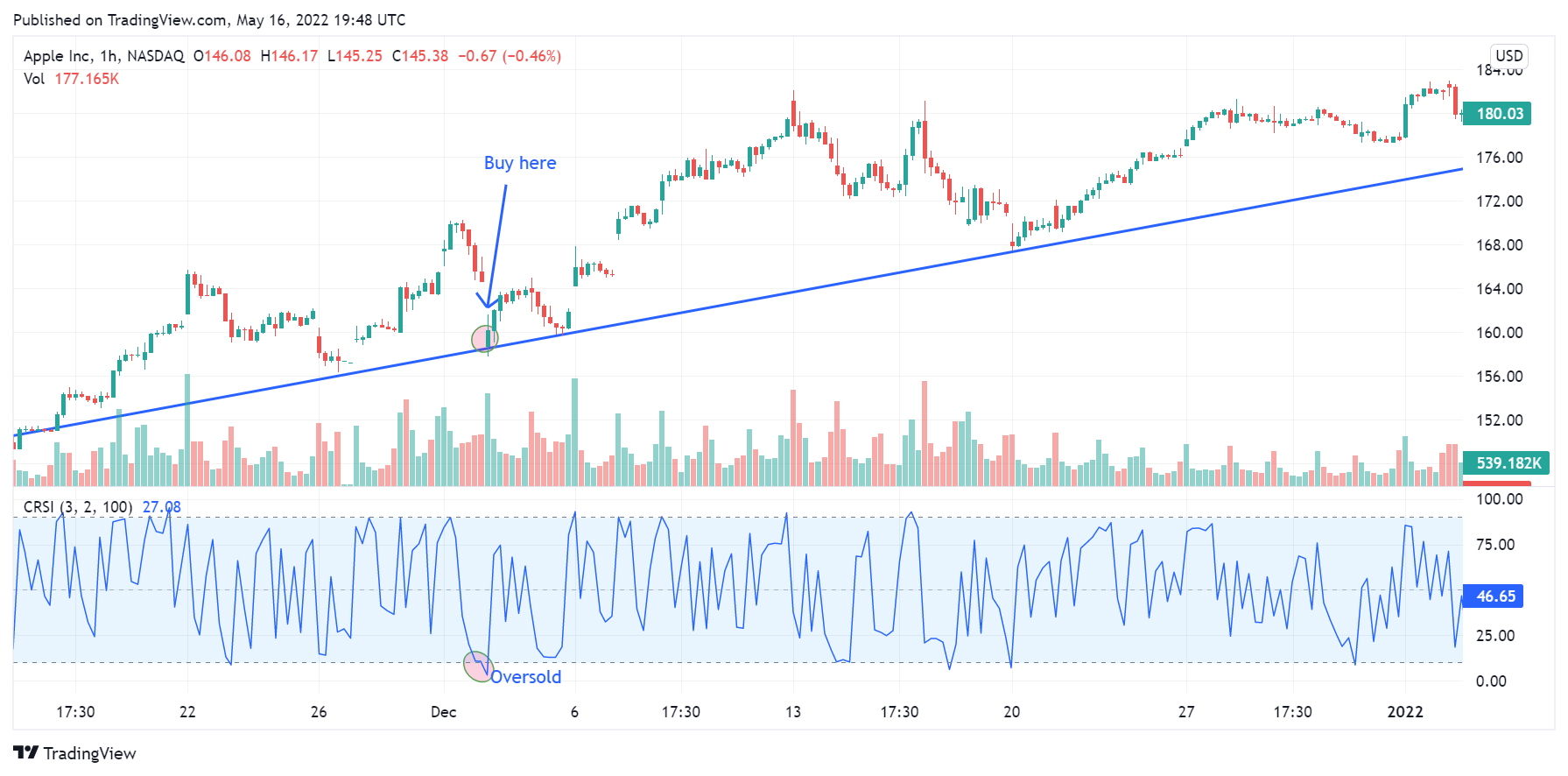This Apple Inc H1 chart shows an up-trending market, as indicated by the upward trend line. Notice that the Connors RSI was in the oversold level when the price pulled back to the trend line.

When the market is trending downward, look to go short. You may follow these steps:

• Wait for a pullback (a temporary price rally) to the descending trendline or a resistance level.
• Check whether the Connors RSI is at your overbought level
• Go short with the next bearish price bar if the indicator is in the overbought region
• Set your profit target at the next support level, or you exit the trade when the indicator reaches the oversold level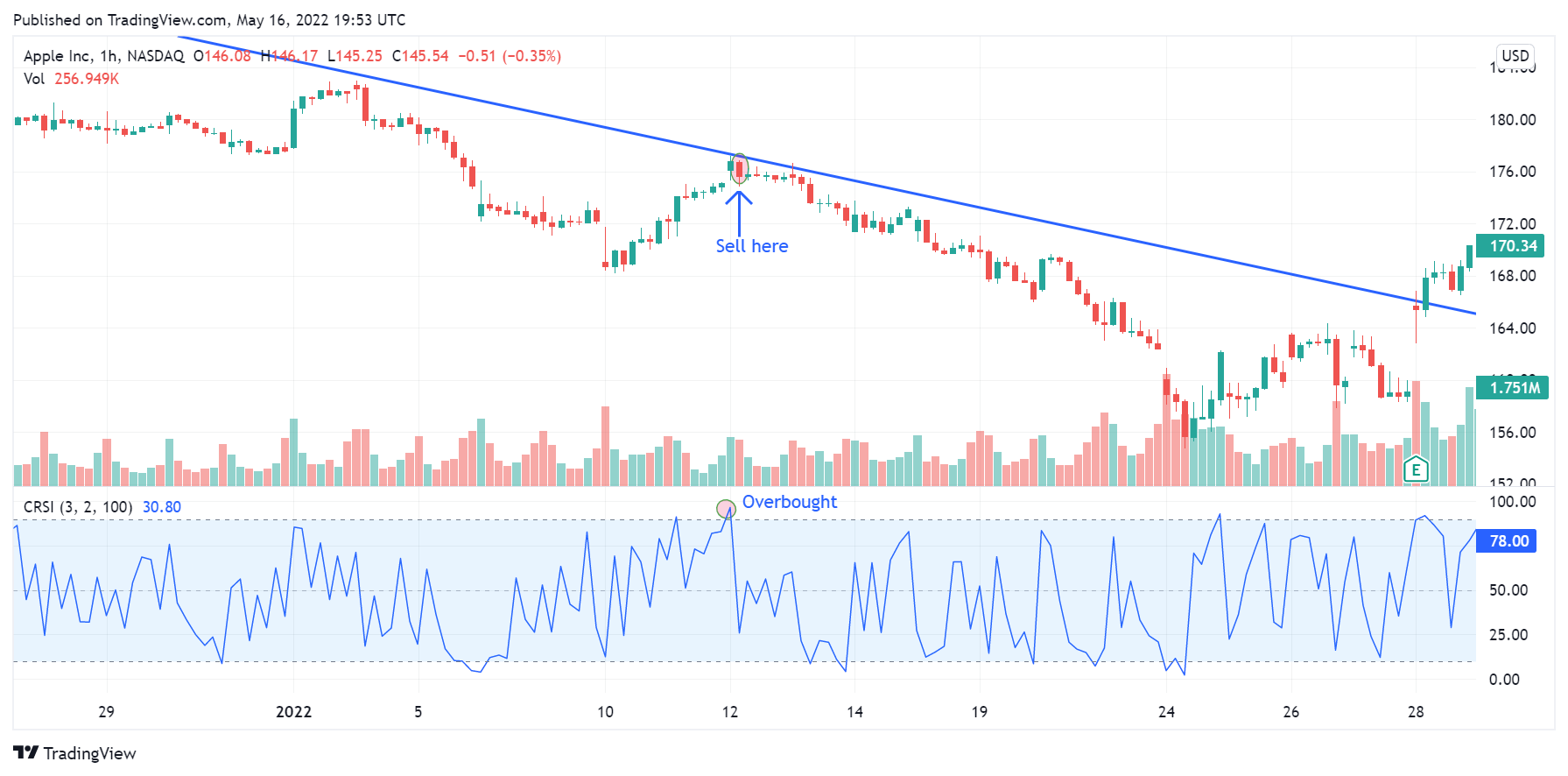Another Apple Inc H1 chart. This one shows a down-trending market, as indicated by the downward trend line. Notice that the Connors RSI was in the overbought level when the price rallied to the trend line.

To use the mean-reversion strategy, you must be sure that the market is in a range-bound situation. There are many ways you can confirm that. One is a flat-lying moving average indicator. Another option is to use the ADX indicator, which must stay below 25. If you are experienced, you can use price action — the price moving sideways and swinging between two levels. Once you have determined that the market is range-bound, here’s what you may do:

• Mark the upper (resistance) and lower (support) boundaries of the price
• Wait for the price to rally to the upper boundary or fall to the lower boundary
• If the price is at the upper boundary, check whether the Connors RSI is overbought, and go short on the next bearish price bar.
• If the price is at the lower boundary, check whether the Connors RSI is in the oversold region, and go long on the next bullish price bar.
• For a long position, set your profit target just below the upper boundary
• For a short position, set your profit target just above the lower boundary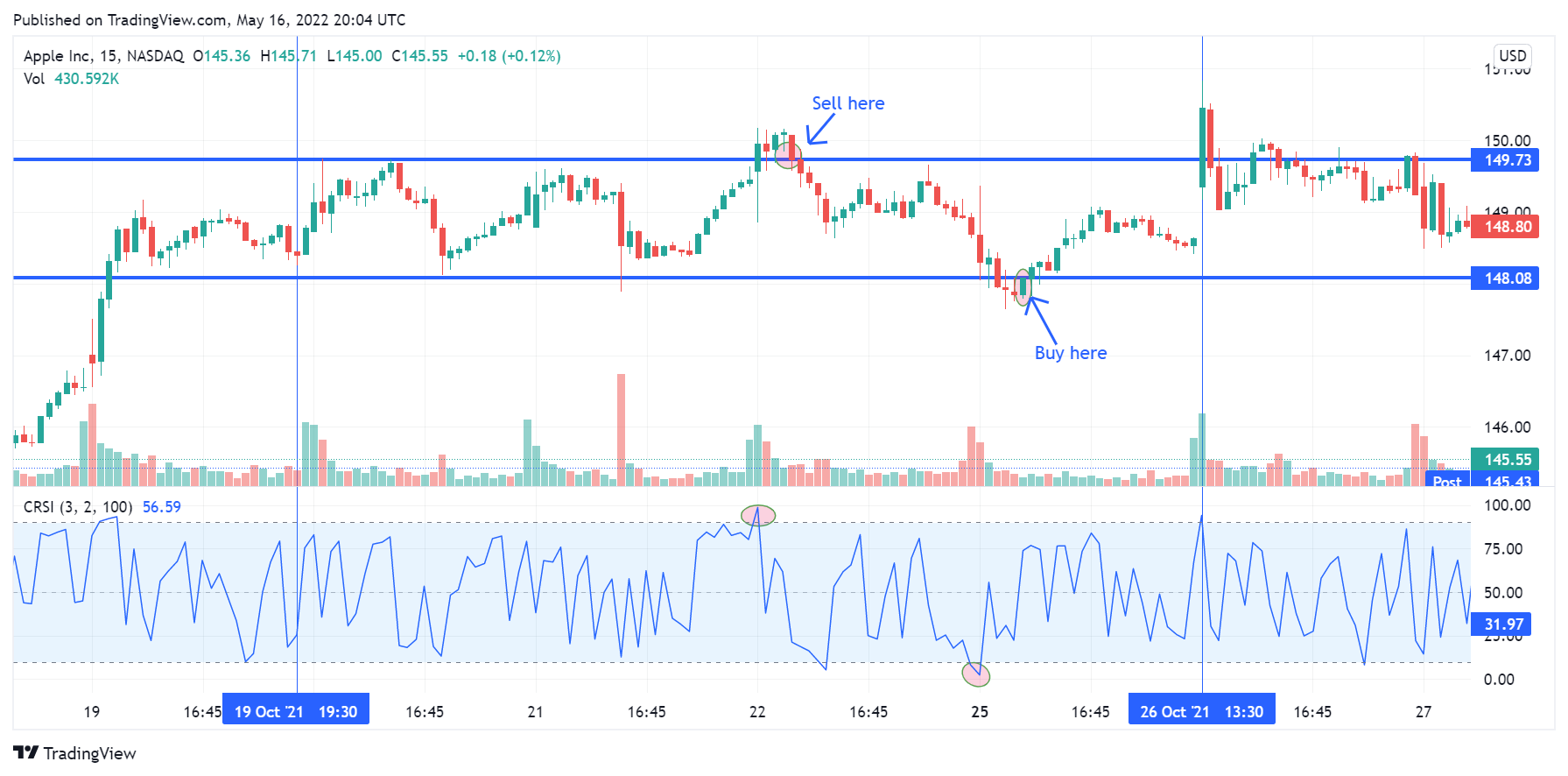A 15-minute Apple Inc chart showing a range-bound market. Note the oversold and overbought signals that formed when the price tested the boundaries of the range.

## Connors RSI vs. the traditional RSI: What is the difference?

The traditional RSI and Connors RSI are similar in that both are momentum oscillators, with values that range between 0 and 100, which can be used to identify overbought and oversold levels. However, they differ in several ways.

The traditional RSI is a simple indicator with a default setting of 14, which makes it react slowly to price movements. On the other hand, the Connors RSI is a composite indicator that uses very short averaging periods (2 and 3). It reacts fast to price movement and is more suitable for short-term trading.

Another important difference is that the traditional RSI typically defines 70 and 30 as the overbought and oversold levels, while Connors RSI typically uses 90 and 10 as the overbought and oversold levels.

Let’s start by looking at the three different RSIs when all use a seven-day lookback period:

The upper is Welles Wilder’s RSI. Just by looking at them visually, we can clearly see that they are different, with Connors RSI significantly different than the others. Connors RSI oscillates a lot! Differences are to be expected because, after all, all three are different formulas.

## Backtest of Connors RSI

There is only one way to find out if Connors RSI is good or bad: we need to backtest. Below we ran multiple backtests of Connors RSI on S&P 500 (SPY):

When we start testing an indicator we start with an optimization. In the table below we have tested different parameters for both the lookback period and the threshold levels for both when to enter and exit. For simplicity, we use the same number of days in all the three parameters needed to calculate Connors RSI (read above to understand all three parameters of Connors RSI).

Our optimization table looks like this:

Let us explain: The marked blue row shows the results of using a two-day RSI and buying when the close crosses below the Connors RSI value of 15 and selling when it goes above 85 (100-35). The column called profit factor shows a value of 2.08 which was the best value with a decent amount of trades (288).

## Larry Connors’ strategies:

We have backtested many of Larry Connors’ strategies:

## Amibroker and Connors RSI

Amibroker doesn’t come with code for Connors RSI. For your convenience we coded it and you can buy it together with all the other code for all our free strategies and articles.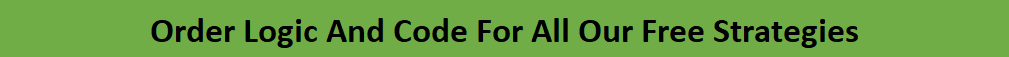## The best settings for Connors RSI

While we only backtested a fraction of the possible trading strategies that can be used for Connors RSI. we believe we can make some general assumptions on the optimization we did above:

• It’s best to use a low number of days. This is because the stock market is mean-revertive in the short term.
• When using a low number of days, it’s best to use a very low threshold – below 20.
• While using a low threshold for entries, it’s better to use a relatively lower threshold for exits: 50-70.

We end the article by showing the equity curve of a specific Connors RSI trading strategy: We use a two-day lookback period, a buy threshold of 10, and we exit when today’s close is higher than yesterday’s high.

This is the equity curve and the drawdowns: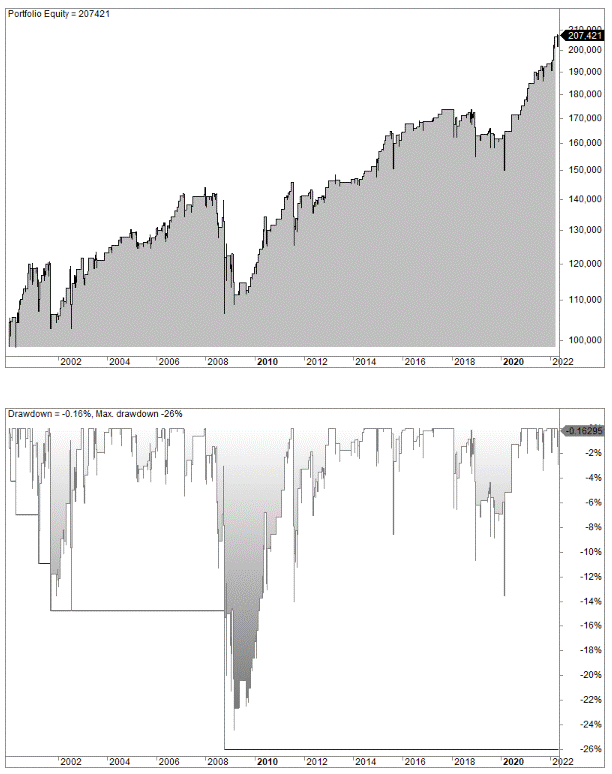The result was not particularly impressive.

Welles Wilder’s RSI is easier to both use and interpret than Connors RSI, thus we like to stick to Wilder’s version.